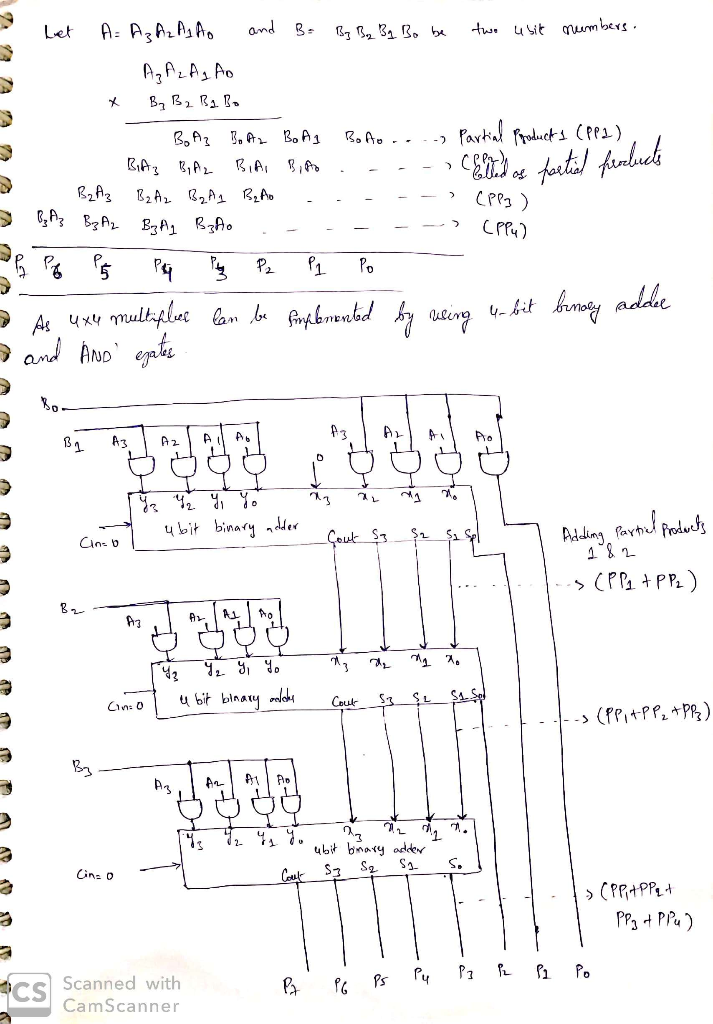##### Question

In: Electrical Engineering

# What's the schematic of the 4-bit multiplier that has two 4-bit inputs and an 8-bit output...

What's the schematic of the 4-bit multiplier that has two 4-bit inputs and an 8-bit output
with pure combinational logic? Can you draw the circuit?

## Solutions

##### Expert SolutionI have impleted the 4 bit multiplier by using the 4 bit full adder and AND gates which are purely combinational. In this implementation we are giving partial product terms as inputs to 4 bit adder at each state and final product is nothing but sum of all four partial products obtained during multiplication of (A3 A2 A1 A0) *(B3 B2 B1 B0). thank you..

## Related Solutions

##### Design and test a 4-bit rotator that has two inputs: A and rotamt, and two outputs...
Design and test a 4-bit rotator that has two inputs: A and rotamt, and two outputs Yleft and Yright using (System) Verilog. The rotation amount (number of bits to be rotated) is given in rotamt and the output of left and right rotation will be in Yleft and Yright, respectively.
##### 3. The lecture notes show a 4-bit by 4-bit multiplier. If the same approach is used...
3. The lecture notes show a 4-bit by 4-bit multiplier. If the same approach is used to make an 8-bit by 8-bit multiplier: a) How many AND gates are required? b) If each AND gate has 1 ns of delay and each adder has 5 ns of delay, what is the total delay for the 8-bit by 8-bit multiplier? c) Can you think of a better way to array the adders to reduce the delay?
##### Design a 4-bit multiplier by using 4 bit full adder and write a verilog code.
Design a 4-bit multiplier by using 4 bit full adder and write a verilog code.
##### design a 4 bit comparator that compares two numbers of 4 bits. the output should be...
design a 4 bit comparator that compares two numbers of 4 bits. the output should be < > or =. however you're only limited to using multiplexers or full adders. any help on how to draw this will be appreciated
##### Write the code to compare two 8-bit buses, a and b. The output signal is agrb...
Write the code to compare two 8-bit buses, a and b. The output signal is agrb for “a greater than b.”
##### Using JK flipflopDesign a multisim schematic for a 4 bit synchronous counter that counts numbers in...
Using JK flipflopDesign a multisim schematic for a 4 bit synchronous counter that counts numbers in Gray code. 4 bit Gray code is as follows: 0000 0001 0011 0010 0110 0111 0101 0100 1100 1101 1111 1110 1010 1001 1000
##### VHDL Code: Design a 16-bit 4-to-1 multiplexer using data-flow implementation style. Data inputs and output should...
VHDL Code: Design a 16-bit 4-to-1 multiplexer using data-flow implementation style. Data inputs and output should be 16-bit vectors. In your test bench, you should include enough number of test cases to show the correctness of your design.
##### 2. A sequential circuit has two pulse inputs, x1 and x2. The output of the circuit...
2. A sequential circuit has two pulse inputs, x1 and x2. The output of the circuit becomes 1 whenever the pulse sequence x1x1x2x2x2x1 is detected. The output then remains 1 for all subsequent x1 pulses until an x2 pulse occurs. (a) Derive a minimal state table describing the circuit operation. (Here you need to define the states and then perform state reduction). (b) Synthesize the circuit using SR latches in the master rank. (Here, you need to make state assignment,...
##### Write a MATLAB function named myaccel that accepts three inputs and has a single output. Inputs:...
Write a MATLAB function named myaccel that accepts three inputs and has a single output. Inputs: a list of time values a list of position values a single time value. Output: a single number which is obtained by numerically differentiating position with respect to time twice (forward difference method) and then interpolating the results based on the third input. Example: time=0:10; position=time.^3; myaccel(time,position,2.8) % should return 22.8
##### Write a program whose inputs are two integers, and whose output is the smallest of the...
Write a program whose inputs are two integers, and whose output is the smallest of the two values. Ex: If the input is: 7 15 output is: 7 passed through command line *in python